# Phase-Locked Loops for Analog Signals

Principles of Phase-Locked Loops

## Introduction

Phase-locked loops (PLLs) for analog signals are ubiquitous in today’s physics and engineering applications. This white paper presents their essential functions and operating principles. It also covers several practical use cases that can be conveniently implemented with Zurich Instruments’ lock-in amplifiers.

Historically, the earliest PLL systems were proposed for the receivers of amplitude-modulated (AM) signals to take advantage of homodyne detection and avoid the undesired image response caused by heterodyne receivers . To perform properly, a homodyne detector requires a local oscillator (LO) with a frequency equal to the carrier frequency of the received signal, meaning that the LO must tightly follow the phase of the incoming carrier. A PLL circuit was then devised to lock the LO phase to the carrier phase and ensure a constant or time-invariant phase relation between the two oscillatory signals.

A PLL is a closed-loop control system with negative feedback that maintains a well-defined phase relation between two periodic signals: its input as a reference and its output as a follower. As a result of phase-locking, the two signals have the same frequency, which enables coherent signal generation and detection across multiple signal sources. In its simplified form, a PLL consists of the following building blocks :

1. Phase detector
2. PID controller
3. Controlled oscillator

As depicted in Figure 1, the PLL receives an input signal, for instance, from a reference source and transfers its phase evolution to a controlled oscillator to generate an output signal that follows the input signal phase.

The phase detector unit measures the phase difference between the two signals. The outcome of phase detection is then compared to a phase setpoint to generate an error signal for the proportional-integral-derivative (PID) controller. Based on the error signal, the PID controller produces a feedback signal to tune the frequency of the controlled oscillator. This way, the controlled oscillator follows the input signal phase and consequently generates a signal at the same frequency as the input reference.

Following a more detailed overview of the PLL building blocks, we describe typical PLL applications and highlight the differences in their implementations based on the basic PLL scheme in Figure 1. We also provide practical tips on setting up a PLL, choosing its key parameters, and characterizing and optimizing its performance. We conclude the white paper with a brief overview of different noise characteristics and models for PLL systems.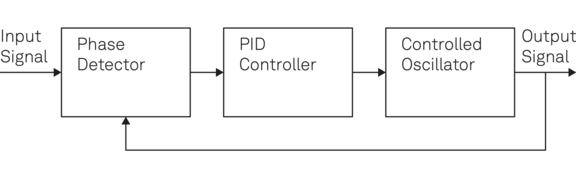Figure 1. Schematic diagram of a PLL showing its basic building blocks. The PLL generates an output signal that follows the phase and frequency of its input signal. It is implemented using a negative-feedback closed loop.

## PLL Building Blocks

PLL systems are designed to deal with analog or digital signals depending on their application. This paper discusses digitally implemented PLLs used for analog signals in advanced research and development experiments. We investigate the case where phase detectors, PID controllers, and reference or controlled oscillators are implemented through digital signal processing.

In particular, our focus is on the Zurich Instruments PLLs, where a field-programmable gate array (FPGA) connects with analog input and output signals via analog-to-digital converters (ADCs) and digital-to-analog converters (DACs), respectively.

### Phase detector

A phase detector takes two periodic signals as inputs and generates an output signal proportional to the relative phase difference between the two input signals. Different techniques are employed to implement phase detection depending on the signal types. For instance, when dealing with square waves used in digital systems, a phase detector is given by an XOR gate followed by a low-pass filter . More generically, dual-phase demodulators are used to obtain the phase difference by measuring the quadrature components X and Y of one input with respect to the other, as illustrated in Figure 2.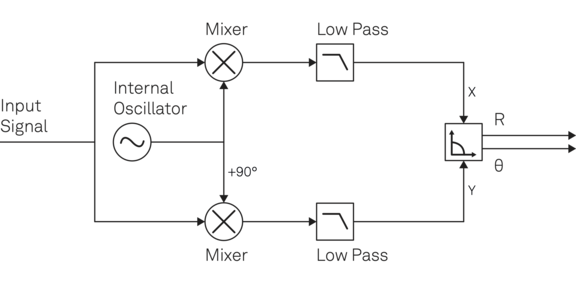Figure 2. Phase detection using dual-phase demodulation of a lock-in amplifier. The in-phase component X and quadrature component Y are generated with two separate signal pathways to derive the relative phase θ and the signal magnitude R of the input signal. The low-pass filters can help to suppress unwanted frequency components and noise.

One of the most common instruments to perform dual-phase demodulation are lock-in amplifiers. Their embedded adjustable low-pass filters provide the additional benefit of noise reduction and the ability to tune the PLL bandwidth, see Figure 2. Low-pass filters can help clean the input signal applied to the PID controller from undesired spectral components and noise in either phase detector signal input. These filters’ cut-off frequency and phase delay set an upper limit on the overall PLL bandwidth. A more detailed description of low-pass filters and their impact on the signal-to-noise ratio (SNR) and measurement speed is provided in .

An additional advantage of using lock-in amplifiers for phase detection is that the lock-in amplifier also measures the signal amplitude. This can be exploited for other control mechanisms such as automatic gain control (AGC), as described in . The amplitude R and the phase θ are easily derived from the measured in-phase X and quadrature Y through a transformation from the Cartesian into the polar coordinates:

$\begin{eqnarray*} \mathrm{R} & = & \mathrm{\sqrt{X^2 + Y^2},} \nonumber\\ \mathrm{\Theta} & = &\mathrm{ atan2\left(Y,X\right)}. \end{eqnarray*}$ (1)

Using the ‘atan2’ function instead of ‘atan’ ensures that the phase angle covers all 4 quadrants of the phase circle, that is, (−π, π], depending on the sign of the quadrature components as plotted in Figure 3.

When the phase signal changes slowly enough compared to the system’s sampling rate, the discontinuity experienced when crossing quadrants can be easily detected and resolved in the digital domain. This function is referred to as ‘phase unwrapping’ and increases the available space of phase values to many multiples of the original interval, as shown in the panels of Figure 4. Zurich Instruments’ lock-in amplifiers support a phase capture range of up to ± 1024 π, which is required, for instance, when stabilizing an optical interferometer over a distance of multiple wavelengths .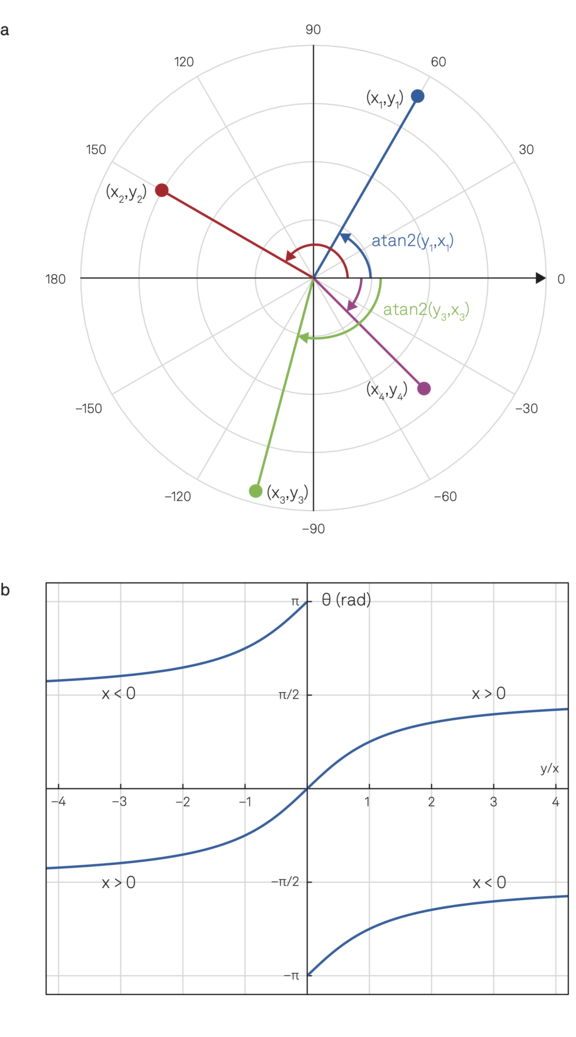Figure 3. (a) Polar coordinates illustrating the phase of a signal component pair (x,y) that covers a range from -180° to +180° represented by atan2(y,x). (b) Depending on the sign of x, atan2(y,x) is a double-valued function versus the ratio y/x returning the angle between the line to the point (x,y) and the positive x axis.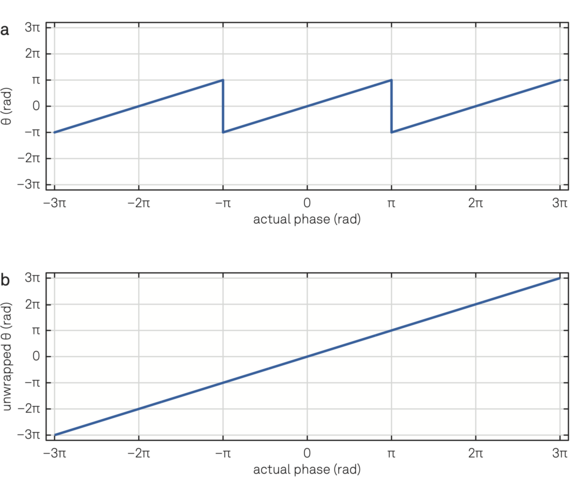Figure 4. (a) Phase measured by a lock-in amplifier used as a phase detector. (b) Unwrapped phase demonstrating a linear relation between the actual and measured phase.

### PID controller

The PID controller receives the phase difference signal from the phase detector and subtracts it from the user-defined setpoint to generate the error signal e(t). The feedback to the controlled oscillator is then generated from the error signal by applying proportional, integral, and derivative operations, as illustrated in Figure 5. Therefore, the final feedback signal u(t) is given by the following expression:

\begin{align*} \mathrm{u(t) = K_0 + K_p e(t) + K_i\int_{0}^{t}{e(\tau)d\tau} + K_d\frac{d}{dt}e(t)}, \label{eqn:PID_controller} \end{align*} (2)

where K0 is the offset value and, Kp, Ki, and Kd are P, I, and D coefficients, respectively. For many practical cases, using the proportional P and the integral component I leads to a sufficiently well-performing and stable operation. The derivative term can be added to further reduce the mean deviation of the error signal from zero. However, this can introduce instability to the loop by enhancing high-frequency components of the error signal. An additional low-pass filter can help use the operation without instability by suppressing the gain of higher frequencies. The PLL speed is characterized by the bandwidth of the closed-loop system. When it comes to tuning the control-loop bandwidth, it is worth taking into consideration a few points:

1. Minimizing the average deviation of the error signal from zero usually requires optimization of the PID parameters to maximize the feedback bandwidth and gain. This often works well in situations where large spontaneous disturbances are rare. Such settings typically lead to a settling time close to its minimum. The Zurich Instruments PLLs come with an Autotune functionality that makes it easier for users to find the optimal working point.
2. High stability and a large capture range for the closed-loop operation are sometimes of higher importance than the mean deviation of the error signal from zero. Such robustness against different kinds of external disturbances is sometimes achieved by using deliberately a weaker and slower feedback signal, and thereby reducing the overreaction and avoiding loss of control. This choice often accompanies a more robust start of the loop filter when initial conditions are not perfectly met. Unfortunately, the optimal settings depend highly on the specifics of the setup and require manual tuning.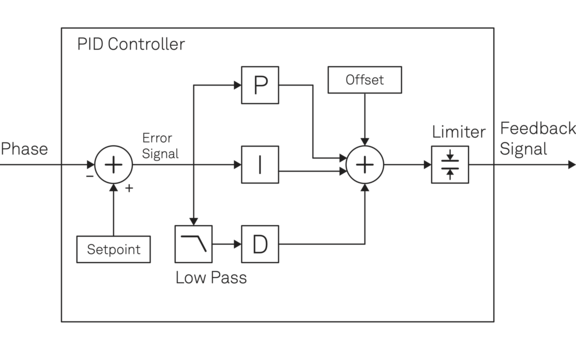Figure 5. Schematic diagram of a PID controller: the phase signal from the phase detector is subtracted from the adjustable phase setpoint and the outcome then passes through the three different branches P, I and D before the resulting signals are added to an offset. A limiter helps restrict the range to a useful parameter space.

1. As only the noise components of the input signal within the loop-filter bandwidth are transferred to the controlled oscillator, it is sometimes necessary to set a well-defined filter bandwidth. Thanks to this powerful filtering it is possible to exclude the leakage of unwanted frequency components to the output signal. The Zurich Instruments PLLs come with a PID Advisor that can model all components involved in the loop and help users quickly identify and set up the required bandwidth.

An additional feature of high practical relevance is an adjustable output offset that makes it possible to start from a default position of the PID controller output so that users can benefit from a good starting point when the loop is turned on. Moreover, setting upper and lower limits for the feedback signal can restrict the control operation to a user-defined frequency range. Setting such limits is particularly helpful when the input signal contains multiple distinct frequency components, but only a specific one needs to be tracked, or when damage to external equipment needs to be avoided.

### Oscillators and frequency references

The controlled oscillator in a PLL is often realized by either a voltage-controlled oscillator (VCO) or a numerically-controlled oscillator (NCO) whose frequencies can be tuned through analog and digital feedback, respectively . VCOs are mainly used as external controlled oscillators, and they are widely-available active components that output a sinusoidal signal with a fixed amplitude, while their frequency can be adjusted over a certain range using an analog input voltage. The main characteristic of a VCO is how much its frequency varies with the change in the control voltage, which ideally follows a linear relationship. In contrast to VCOs, linearity is always assured for NCOs, and they also provide the benefit of the frequency values being available in a digital form at any given time, adding the functionality of a frequency counter. Generally, there are many more ways to produce controllable periodic signals. However, most cases can be traced back to one of the two examples above when it comes to the basic functionality. For instance, the beat note between a laser beam and a frequency comb typically creates a radio frequency signal at the output of a photodiode. When applying a feedback signal to the modulation input of the laser, its optical frequency will alter according to the voltage signal, and as a result, the frequency detected by the photodiode will change. Neglecting all optical signals involved, this is a very similar configuration to using a VCO, and it can be used, for instance, to establish an optical PLL for laser frequency stabilization.

When using Zurich Instruments’ Lock-in Amplifiers, one input to the phase detector always comes from an internal digital oscillator. The frequency of these NCOs, implemented on an FPGA, can be adjusted by setting the frequency value digitally while phase-continuous output, i.e., a steady signal, is assured. The maximum rate at which the NCO frequency can be changed is given by the FPGA clock speed or the frequency register update rate. This rate is much higher than the maximum achievable control-loop bandwidth. Depending on the PLL configuration, the internal NCO can be a leader, a follower, or an intermediate oscillator.

If the NCO is following an external frequency reference, the frequency of the internal NCO is numerically adjusted by the PID controller to keep track of the reference signal. However, if the internal oscillator provides the frequency reference for an external VCO, its frequency is fixed at a specific value while the PID controller applies the feedback signal to adjust the frequency of the external VCO. In case two external sources need to be locked, two PLLs have to be setup with a joint internal NCO serving as an intermediate transmission gear.

It is worth mentioning that accurate and precise periodic signal generation within a wide frequency range is essential for various test and measurement (T&M) applications. Many T&M instruments that cover a broad frequency range use PLL-based frequency synthesis to generate frequency-adjustable periodic signals. To combine the flexibility of wide-range and precise frequency generation with high stability and accuracy, PLLs can integrate the best properties of highly accurate and low phase-noise oscillators, like oven-controlled crystal oscillators (OCXO), with the flexibility of controlled oscillators like VCOs. To do so, a PLL synchronizes the controlled oscillator frequency f with a fraction of the OCXO frequency fREF, serving as a reference.

By making the coefficient factor adjustable, a wide frequency range according to f = (m/n) fREF, see Figure 6, is accessible. The oscillator frequency can now be widely tuned by numerically adjusting the ratio m/n while the OCXO frequency is fixed at a stable frequency, for instance, 10 MHz or 100 MHz in many T&M instruments. In addition to frequency accuracy and stability, the implementation details of the PLL influences also other factors such as phase noise, spur sidebands, and lock-time which determine the quality of the generated signal and thus influence the performance of systems in which such frequency synthesis is deployed. For most digital signal processing instruments, this functionality is built-in and thus the signal of the internal NCOs serves as a reliable frequency reference.

## Applications

The main functionality of a phase-locked loop is the timebase synchronization of two systems mainly represented by two oscillators. There exist numerous applications where two or more oscillatory signals need to be synchronized. While PLLs are extensively used in digital systems for operations such as jitter reduction, skew suppression, frequency synthesis, and clock recovery, here we focus on the applications of PLLs for analog signals, In particular, those belonging to one of the following three configurations:

1. Frequency tracking: the PLL maps an external source to an internal oscillator, see Figure 6.
2. Resonance driving: the PLL drives the time-varying resonance of a device, see Figure 9.
3. Oscillator control: the PLL provides analog feedback to an external variable-frequency source, see Figure 10.

### Frequency tracking

A broad range of applications in which lock-in amplifiers, transmission- and embedded-systems are used require coherent signal detection where synchronization of the signal source and the signal detector is needed. In its most general form, illustrated in Figure 6, a frequency tracking system receives an external reference signal and maps its frequency content to the internal oscillator of the system. A concrete example of this application is the homodyne detection by a lock-in amplifier with an external reference. To properly recover the amplitude and phase of an input signal, a lock-in amplifier requires an external reference signal from the signal generator, which determines the measurement frequency, for instance, an optical chopper. The lock-in amplifier then needs to lock its internal oscillator to that external reference frequency to coherently detect the received signal of interest. This is typically done by a PLL configuration similar to Figure 6. Finding the right center frequency and bandwidth of the PLL is usually automated for most use cases. However, when specific bandwidth requirements are given or the spectrum contains multiple components, adjusting individual PLL settings may be required to ensure accurate frequency tracking. In addition, this mapping of an external reference signal to an internal oscillator by using a defined bandwidth leads to a jitter- and spurious-free signal at the tracked frequency. This aspect can be beneficial for applications that require spectral filtering, where one can choose a deliberately low closed-loop bandwidth to exclude or suppress unwanted noise sources of the external signal.

Another advantage of an all-digital implementation is the possibility of multiplying or dividing the tracked frequency to generate an internal reference at its harmonics and ratios without losing any signal quality. Using fractional multiples of the reference frequency for demodulation enables additional measurement schemes based on the same external reference, for instance, optical chopping with harmonic-blade wheels.

Another important application of the PLL configuration in Figure 6 is carrier recovery. Coherent demodulation of received signals in a transmission system requires the receiver to know the frequency of the carrier wave. However, because of the distance between the transmitter and receiver they cannot share the same clock source and thus perfect synchronization is unachievable unless the receiver uses a PLL to lock to the carrier sent by the transmitter. In addition to the modulated signal, many communication systems send a pure tone as a pilot to which the receiver’s PLL can lock. Without carrier recovery by a PLL unit, coherent communication cannot be achieved in most of transceiver systems. In case no pilot is sent or the modulated signal is carrier-suppressed, slightly modified PLL configurations, e.g., Costas loops, are utilized to extract the carrier frequency . Similarly for lock- in amplifiers, when a distinct external reference is not available, it might be possible to lock the internal oscillator to the measurement signal itself depending on the signal quality. This so-called ‘auto-reference’ configuration of lock-in amplifiers can only extract the amplitude information of the signal and not the phase.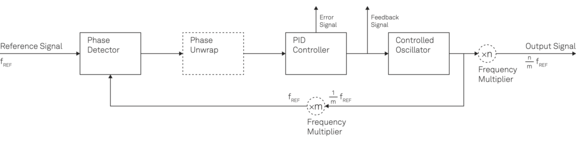Figure 6. Schematic diagram showing the building blocks of a PLL with extended functionality for phase unwrap and fractional frequency locking. Besides the output signal, many applications benefit from intermediate signals such as error and feedback signals.

Frequency extraction, filtering and counting
Similar to the example above, PLLs can also extract specific frequency components from signals that hold multiple distinct frequencies. This extraction requires tuning the variable source close to the desired frequency at PLL start-up to transfer the right spectral component to the PLL internal oscillator. This method is very powerful and flexible to filter out specific spectral components, track them and reuse them as pure signals in other parts of the setup. Given that the feedback to the internal NCO is digital, the instantaneous frequency can be tracked and logged at a high rate, which then offers an extra frequency counter functionality.

FM demodulation
Frequency modulation (FM) is a technique to encode the information of a message signal into the frequency of a carrier wave. It is widespread in analog and digital systems because of its resilience to amplitude noise and fluctuations. In FM systems, the instantaneous frequency of the carrier follows the time variation of the message signal. Therefore, a frequency tracker is required to demodulate the carrier wave and extract the message from the carrier frequency. Among the demodulation approaches for FM systems, the PLL stands out with low distortion and reduced sensitivity to amplitude noise.

As shown in Figure 7, a PLL-based FM demodulator is relatively straightforward and does not require changes in the basic PLL configuration. When no modulation is applied to the carrier wave, the feedback signal applied to the controlled oscillator is in the middle of its range to generate the carrier frequency. However, if the modulation changes the carrier frequency, the loop filter alters the feedback voltage applied to the oscillator to change its frequency. This way, the closed loop remains locked, i.e., the oscillator signal follows the phase of the received FM signal. It can be seen that the feedback signal applied to the controlled oscillator is proportional to the frequency change of the received carrier. Therefore, the demodulated signal can be amplified and delivered to the next stage for acquisition and processing.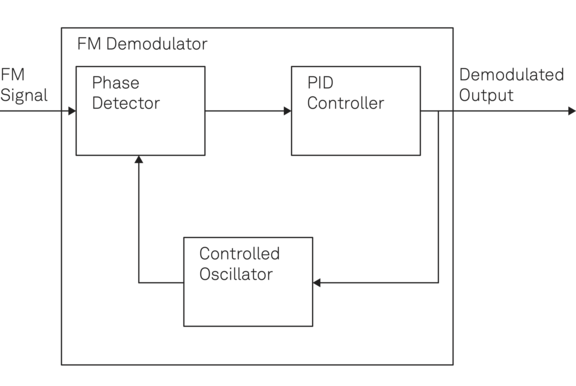Figure 7. Schematic diagram of a PLL-based FM demodulator where the output of demodulation is proportional to the feedback signal applied to the controlled oscillator.

The primary consideration in designing a PLL-based FM demodulator is the demodulation bandwidth determined by the PLL’̇s closed-loop bandwidth. The demodulation bandwidth determines the signal bandwidth and thus the maximum amount of information that can be transmitted over the communication channel per time. Hence, the phase detector and the PID controller must be fast enough to cover the required bandwidth of the FM signal. Moreover, using an oscillator with a highly linear response is crucial to minimize the distortion of the demodulated signal and keep the demodulation process as linear as possible. In other words, when using VCOs as controlled oscillators, the voltage to frequency curve needs to be as linear as possible within the frequency range of the received FM signal.

### Resonance driving

Driving a device with controlled phase and frequency is instrumental in many applications such as atomic force microscopy (AFM) and micro- and nano-electromechanical systems (MEMS/NEMS). In such applications, a part of the system behaves like a resonator with a Lorentzian-shape amplitude transfer function and a sigmoid-shape phase evolution similar to Figure 8, illustrating the Bode magnitude and phase plots of a resonator with a resonance frequency of 1.84 MHz. One can benefit from resonance enhancement and linearization of the measurement response by driving the device at resonance. Typically, the resonance frequency of such systems varies with some physical quantities like temperature, force, etc. Therefore, to drive the resonator at a fixed phase, it is required to adjust the signal frequency according to the resonance frequency variations due to the environmental parameters. A PLL which controls the drive frequency in a way that the output phase of the resonator remains at a user-defined setpoint is able to achieve such resonant driving. Figure 9 illustrates the block diagram of a PLL-controlled resonator drive in which the oscillator provides the driving signal for the resonator and the reference signal for the phase detector. The PLL assures the device is continuously driven at the same working point even when its resonance frequency changes with time.

The PID controller translates the error signal at the phase detector output to a control signal at the oscillator input to adjust the signal frequency according to the variations in the resonator response.

As an example, the cantilever head of an AFM includes a tuning-fork resonator which measures the force field exerted by the surface of the sample under test using the induced shift in its resonance frequency. To measure this frequency shift and thus characterize the surface topography, a PLL is required to lock to the cantilever’s resonance. The PLL error signal will then represent the sample surface. Another typical example is inertial measurement systems such as MEMS gyroscopes and accelerometers . It is crucial to drive the vibrational mass of such a system at its resonance regardless of the rotational motion it observes. Since rotation alters the resonance frequency, a PLL is essential for the stable drive of the sensor at its varying resonance frequency. This application of PLLs in controlling resonance systems includes many more examples such as pump-probe, ion trapping, and parametric feedback cooling experiments, where the PLL is modified to adapt for the application-specific requirements. One example is the out-of-phase driving of the system at the second harmonic of the resonance frequency in parametric feedback cooling .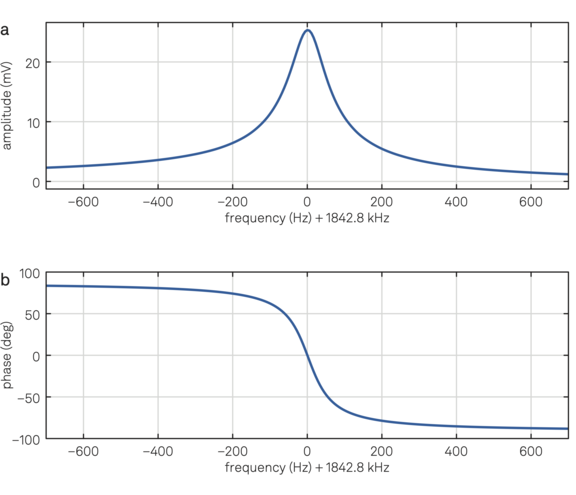Figure 8. (a) Bode magnitude and (b) phase plots of a crystal resonator measured by the frequency response analyzer of a Zurich Instruments lock-in amplifier.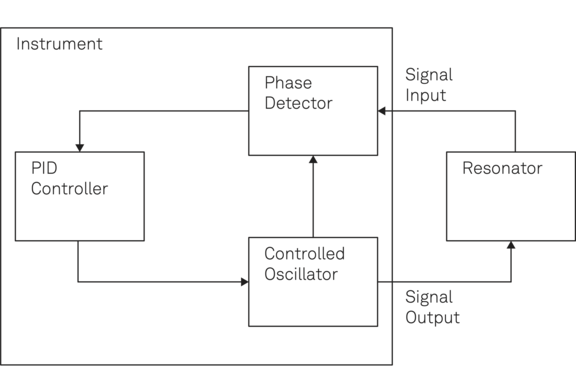Figure 9. Schematic diagram showing the closed-loop control of a resonator by means of a PLL. The resonator together with the instrument form a PLL system where the controlled oscillator follows the varying resonance frequency by keeping the relative phase constant.

### Oscillator control

As mentioned before and for simplicity, we model any external oscillator to be controlled as a VCO. In the PLL configuration sketched in Figure 10, the internal oscillator provides the reference signal for the phase detector measuring the phase of the external oscillator relative to the reference. The PID controller converts the measured phase difference into a feedback signal and applies it to the external VCO to adjust its output frequency. This way, one can map the timebase content of a stable internal NCO, to an external system. The spectral filtering of the phase detector along with the programmable output limiter of the analog voltage signal contributes to a stable operation at the desired frequency, even after re-locking in case the lock was lost. Also, the system is not driven at any values outside the defined safe zone where reliable operation is guaranteed even if the integrator goes into saturation.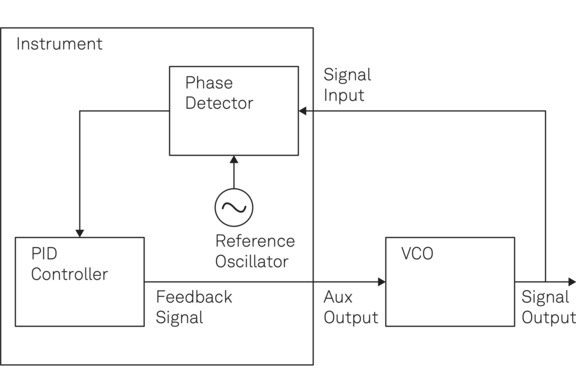Figure 10. Controlling the frequency of a VCO based on a reference oscillator using a phase-locked loop.

## Starting up a PLL

The following steps provide a systematic approach to successfully closing a feedback loop and getting a PLL operating:

1. Obtain the open-loop response of the system.
2. Find a coarse and conservative set of PID parameters to start the loop.
3. Tune the PID parameters to optimize for SNR, speed, or robustness.

Finding a suitable initial set of PID parameters and starting conditions can be tricky. LabOne®, the Zurich Instruments control software to interface with the instrument hardware, provides effective tools for all the 3 steps to make the time-consuming process of starting up and optimizing a PLL as efficient and easy as possible. As a first step, one needs to obtain the open-loop response of the device under test (DUT). This can be the spectral response of a resonator or the curve displaying the frequency with respect to the tuning voltage of a VCO. The sweeper module of LabOne offers all the ingredients required to obtain the open-loop response of a system as it can sweep the frequency, phase, amplitude, and offset of the applied signals and acquire the measured outcome. Moreover, the integrated mathematical tools such as curve fitting, tracking, peak-trough finding, etc. help the user extract the system characteristics such as quality factor, gain, and slope needed for proper PID controller tuning.

Once the system response is known, a specific target bandwidth can be set by taking into account the characteristics of the low-pass filter used in the phase detector unit as well as the system delays introduced at various points.

Conveniently, LabOne offers a PID Advisor that employs an optimization algorithm to tune the PID controller and determine the required filter settings for a given target PLL bandwidth. The PID Advisor takes into account all physical delays and gains of the hardware. Moreover, it features a variety of mathematical models to factor in the transfer function of the attached device. After selecting the device configuration, whether it is an internal PLL, an external resonator, or a VCO-type configuration, the Advisor runs its algorithm to calculate the P, I, and D parameters, and obtain the filter characteristics as well as the phase margin and actual bandwidth. It also displays various temporal and spectral figures such as Bode magnitude and phase plots plus the step response at various entry points of the entire system, which illustrate how fast and stable the designed PLL works.

Applying the PID settings obtained by the PID Advisor to the instrument and enabling the PID controller should close the PLL successfully. Using the plotter tool of LabOne, one can continuously monitor the PID error and output signals and at the same time adjust the PID settings and observe its impact on the PLL performance. In addition to manual adjustment of PID parameters, the Autotune feature of the PID controller available in LabOne can modify the PID settings automatically to improve the PLL performance. Figure 11 shows the PID/PLL tab of LabOne user interface, where all the required tools for designing, optimizing, and running a PLL are provided in a user-friendly web environment.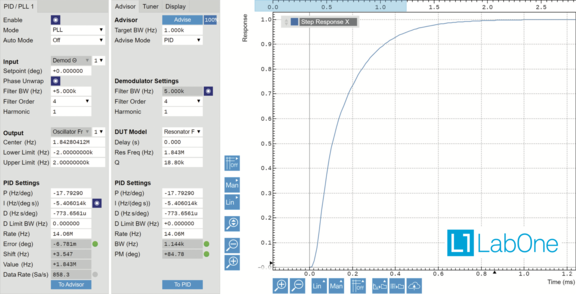Figure 11. PLL/PID controller tab of LabOne user interface showing the input, output, phase unwrap, and PID parameters (left), PID Advisor, Tuner, and DUT model (middle), and the step response of the final closed-loop system (right).

## Noise in PLLs

The analog output of a PLL is a sinusoidal signal with a fixed amplitude but varying frequency and phase following a setpoint or a reference signal. In an ideal PLL with a fixed setpoint, the frequency and phase of the output signal should be time invariant. However, due to the noise in real systems a PLL suffers from phase and frequency fluctuations. The phase fluctuation can be modeled by a zero-mean random process φ(t) with a standard deviation of σφ. Therefore, the output of a PLL with a fixed setpoint is given by the following sine signal at the carrier frequency fc in Hz and with the phase ψ in rad:

\begin{align*} \mathrm{z(t) = A\sin(2\pi f_c t + \psi + \phi(t)),} \end{align*} (3)

where A is a fixed amplitude. The phase noise is best represented by its power spectral density (PSD) denoted by Sφ(f), which is obtained by taking the Fourier transform from the auto-correlation of φ(t). The phase noise PSD demonstrates how much noise power exists in a unit bandwidth of 1 Hz at an offset frequency f away from the carrier frequency fc. Its unit is rad2/Hz; however, in many applications, it is also expressed in dBc/Hz, which is relative to the carrier power. Here, we explain how to express the phase noise in different units. The IEEE standard  defines Sφ(f) in rad2/Hz as the one-sided (double sideband or DSB) spectral density of phase and introduces the two-sided (single sideband or SSB) spectral density Lφ(f) as a standard way of representing phase noise in dBc/Hz:

\begin{align*} \mathrm{\mathrm{\mathcal{L}_\phi(f) = \frac{S_\phi(f)}{2}}.} \end{align*} (4)Therefore, the phase noise PSD provided in data sheets is always 3 dB below the actual one-sided spectrum; care should be taken when calculating the noise power and temporal jitter based on the spectral density.

In other words, the phase noise power σφ2 within a measurement bandwidth δf, expressed in rad2, is obtained by either of these two integrals:

\begin{align*} \mathrm{\sigma_{\phi}^2 = \int_{\delta f}{S_\phi(f)df} = 2\int_{\delta f}{\mathcal{L}_\phi(f)df}.} \end{align*} (5)

For example, a PLL with a constant SSB phase noise of −120 dBc/Hz around a certain offset frequency suffers from a phase fluctuation of $$\mathrm{\sqrt{2\times10^{-120/10}\times5}=3.16}$$ μrad caused by a 5-Hz measurement bandwidth around that offset frequency.

According to the fundamental relation between frequency and phase, the frequency fluctuation ν(t) of the PLL output in Hz is obtained from its phase noise using the following expression:

\begin{align*} \mathrm{\nu(t) = \frac{1}{2\pi}\frac{d}{dt}\phi(t).} \end{align*} (6)

Similarly to the phase noise PSD, we can define the power spectral density of frequency noise as the Fourier transform of its auto-correlation. The frequency noise PSD, denoted by Sν(f), is expressed in Hz2/Hz and it can be obtained from the phase noise PSD using the equation below:

\begin{align*} \mathrm{S_\nu(f) = f^2 S_\phi(f).} \end{align*} (7)

Depending on the application, one may be interested in either phase or frequency noise; using Eq. 7 makes it possible to convert one PSD into another.

### Power law

A heuristic approach to describe the power spectral density of phase and frequency noise is to use a power-law function as follows :

\begin{align*} \mathrm{S_\phi(f) = b_0 + \frac{b_1}{f} + \frac{b_2}{f^2} + \frac{b_3}{f^3} + \frac{b_4}{f^4}.} \end{align*} (8)

Each term in the above expression corresponds to a different type or color of noise. For instance, b1 shows the contribution of the flicker phase noise in the spectral density, which is determined by a slope of −10 dB/decade, while b2 corresponds to the Brownian phase noise with a slope of −20 dB/decade in the noise spectrum. According to Eq. 7, the frequency noise spectrum can be described using a power-law function as follows:

\begin{align*} \mathrm{S_\nu(f) = b_0 f^2 + b_1 f + b_2 + \frac{b_3}{f} + \frac{b_4}{f^2}.} \end{align*} (9)

Table 1 shows different colors of phase and frequency noise contributing to the overall noise spectral density of a PLL. Each color determines the slope of the spectrum on a logarithmic scale. Indeed, the term fi in the table corresponds to a noise color with a slope of 10i dB/decade in the spectral density. Figures 12 and 13 depict the phase and frequency noise spectrum of an oscillator controlled by a PLL. Depending on the components of a PLL system, one of the noise colors can be dominant in a certain frequency range. This can be understood from the slope of the spectrum curve in that specific frequency range.

For example, the phase noise is almost white for frequencies above 1 kHz in the case shown in Figure 12 as the spectrum curve is approximately flat for this frequency range. As a frequency-domain tool, the power-law description of phase noise links tightly to the Allan variance or the Allan deviation, which is a time-domain tool to analyze the noise characteristics of an oscillator. By measuring the spectrum or the Allan variance, we can easily obtain the other one and provide a full description of phase and frequency noise in the time- and frequency-domain .

Noise type Sφ(f) Sν(f)
White phase noise
Violet frequency noise
$$\mathrm{b_0}$$ $$\mathrm{b_0 f^2}$$
Flicker (pink) phase noise
Blue frequency noise

$$\mathrm{\frac{b_1}{f}}$$

$$\mathrm{b_1 f}$$
Brownian (red) phase noise
White frequency noise
$$\mathrm{\frac{b_2}{f^2}}$$ $$\mathrm{b_2}$$
Flicker frequency noise $$\mathrm{\frac{b_3}{f^3}}$$ $$\mathrm{\frac{b_3}{f}}$$
Brownian frequency noise $$\mathrm{\frac{b_4}{f^4}}$$ $$\mathrm{\frac{b_4}{f^2}}$$

Table 1. Various types of phase and frequency noise and their contribution to the noise spectrum.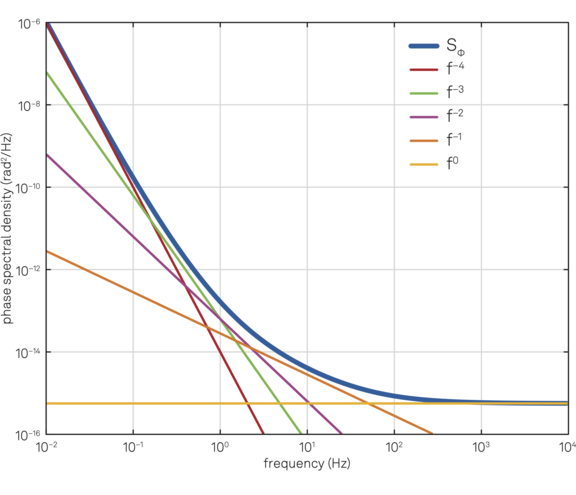Figure 12. Phase noise spectral density and the contribution of different noise colors.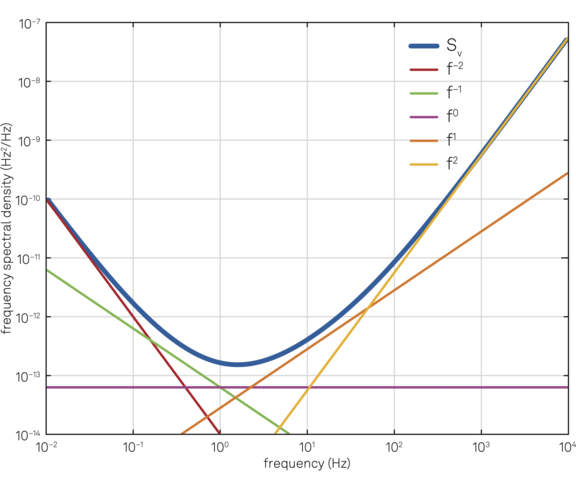Figure 13. Frequency noise spectral density and the contribution of different noise colors.

### Leeson effect

A common scenario in resonance tracking is given by a resonator driven by a PLL in which the white phase noise of its VCO is dominant. As a result, we expect a frequency noise of order f2. However, in addition to this violet frequency noise, we see a white frequency noise term that can be explained by the Leeson effect . Suppose a resonator with a resonance frequency f0 and a quality factor Q is driven by the VCO of a PLL. According to the Leeson effect, the phase noise Sφ at the resonator output can be obtained from the VCO phase noise Sψ at the input of resonator by the following expression :

\begin{align*} \mathrm{S_\phi(f) = \bigg(1+\frac{f_L^2}{f^2}\bigg)S_\psi(f),} \end{align*} (10)

where fL =f0/2Q is called the Leeson frequency. If the VCO has a white phase noise of b0, then according to Eq. 10 the phase noise of resonator includes a white term b0 and a red term b0fL2/f2. Applying Equation 7 to the resonator phase noise leads to a frequency noise spectrum with two terms: a blue term b0f2 and a white term b0fL2. Figure 14 illustrates the spectrum of a resonator’s phase and frequency noise when it is driven by a VCO with white phase noise. The figure clearly shows that, for frequencies above the Leeson frequency of the resonator (in this case fL = 20 Hz), the dominant noise is from the VCO; by contrast, for frequencies below fL the resonator contributes to the noise spectrum and modifies the noise color.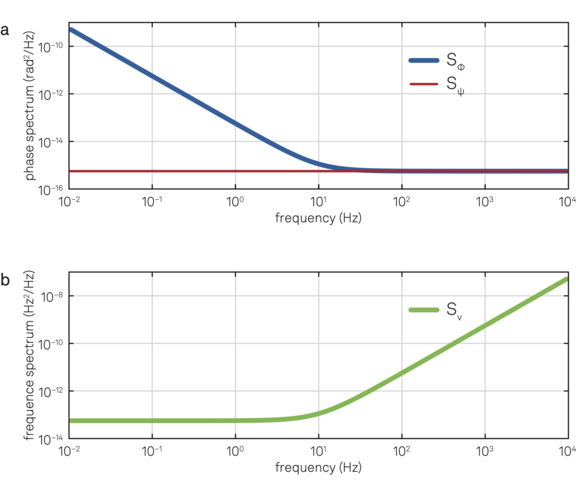Figure 14. (a) Phase noise of a resonator (blue line) with a Leeson frequency of 20 Hz driven by a controlled oscillator with white phase noise (red line). (b) Frequency noise of the resonator.

## Conclusion

As an essential component for phase and/or frequency tracking and synchronization, phase-locked loops are widely used in physics, electronics, photonics, and communications. A PLL includes three main components: a phase detector, a PID controller, and a controlled oscillator. Each of these building blocks contributes to the overall response of the entire system.

The most common PLL applications can be implemented with one of these three main configurations: frequency tracking, resonance driving, and oscillator control. FPGA-based phase-locked loops for analog signals such as those offered with Zurich Instruments’ lock-in amplifiers provide closed-loop frequency and phase control with integrated features such as phase unwrapping and autotune functionality, as well as user-friendly tools, including the PID Advisor and parametric sweeper.

## References

 Thomas H. Lee. The Design of CMOS Radio Frequency Integrated Circuits, 2nd ed. Cambridge University Press, 2003.
 Roland E. Best. Phase-Locked Loops. McGraw Hill, 2003.
 Behzad Razavi. Design of Analog CMOS Integrated Circuits. McGraw Hill, 2002.
 Zurich Instruments. Principles of Lock-in Detection, 2016.
 Zurich Instruments. Control of MEMS Coriolis Vibratory Gyroscopes, 2015.
 Donald R. Stephens. Phase-Locked Loops for Wireless Communications: Digital, Analog and Optical Implementations. Wiley, 2002.
 J.P. Costas. Synchronous communications. Proceedings of the IEEE, 90(8):1461–1466, 2002.
 Zurich Instruments. Parametric Feedback Cooling, 2014.
 IEEE standard definitions of physical quantities for fundamental frequency and time metrology--random instabilities. IEEE Std. Std 1139-2008, 2009.
 Enrico Rubiola. Phase Noise and Frequency Stability in Oscillators. Cambridge University Press, 2010.
 D. B. Leeson. A simple model for feedback oscillator noise. Proceedings of the IEEE, 54(2), 1966.